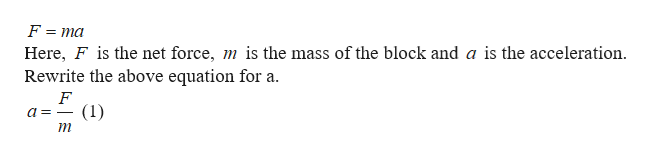# If the mass of a sliding block is tripled while a constant net force is applied, by how much does the acceleration decrease?

Question
39 views

If the mass of a sliding block is tripled while a constant net force is applied, by how much does the acceleration decrease?

check_circle

Step 1

The equation for the net ...help_outlineImage TranscriptioncloseF ma Here, F is the net force, m is the mass of the block and a is the acceleration. Rewrite the above equation for a (1) m fullscreen

### Want to see the full answer?

See Solution

#### Want to see this answer and more?

Solutions are written by subject experts who are available 24/7. Questions are typically answered within 1 hour.*

See Solution
*Response times may vary by subject and question.
Tagged in
SciencePhysics

### Newtons Laws of Motion